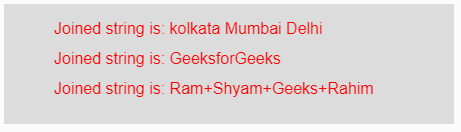Open in App
Not now

# p5.js | join() function

• Difficulty Level : Medium
• Last Updated : 12 Apr, 2019

The join() function in p5.js is used to join the input array of strings into a single string using separator. This separator might be any character which gets their position in between two strings of the input array.

Syntax:

`join(Array, Separator)`

Parameters: This function accepts two parameters as mentioned above and described below:

• Array: This is the array of strings which are to be joined.
• Separator: This is any character to be placed in between two strings of the input array.

Return Value: It returns the joined strings.

Below programs illustrate the join() function in p5.js:

Example 1: This example uses join() function to join the input array elements.

 `function` `setup() {  ` `  `  `    ``// Creating Canvas size ` `    ``createCanvas(450, 120);  ` `}  ` `  `  `function` `draw() {  ` `      `  `    ``// Set the background color  ` `    ``background(220);  ` `    `  `    ``// Initializing the Array of strings ` `    ``let Array1 = [``"Geeks"``, ``"for"``, ``"Geeks"``]; ` `    ``let Array2 = [``"1"``, ``"2"``, ``"3"``]; ` `    ``let Array3 = [``"a"``, ``"A"``, ``"b"``, ``"B"``]; ` `    `  `    ``// Initializing the separator character ` `    ``let Separator1 = ``"/"``; ` `    ``let Separator2 = ``"&"``; ` `    ``let Separator3 = ``"*"``; ` `          `  `    `  `    ``// Calling to join() function. ` `    ``let A = join(Array1, Separator1); ` `    ``let B = join(Array2, Separator2); ` `    ``let C = join(Array3, Separator3); ` `          `  `    ``// Set the size of text  ` `    ``textSize(16);  ` `      `  `    ``// Set the text color  ` `    ``fill(color(``'red'``));  ` `    `  `    ``// Getting joined strings ` `    ``text(``"Joined string is: "` `+ A, 50, 30); ` `    ``text(``"Joined string is: "` `+ B, 50, 60); ` `    ``text(``"Joined string is: "` `+ C, 50, 90); ` `       `  `}  `

Output:Example 2: This example uses join() function to join the input array elements.

 `function` `setup() {  ` `  `  `    ``// Creating Canvas size ` `    ``createCanvas(450, 120);  ` `}  ` `  `  `function` `draw() {  ` `      `  `    ``// Set the background color  ` `    ``background(220); ` `          `  `    ``// Calling join() function over the parameter ` `    ``// Array of strings and separator ` `    ``let A = join([``"kolkata"``, ``"Mumbai"``, ``"Delhi"``], ``" "``); ` `    ``let B = join([``"Geeks"``, ``"for"``, ``"Geeks"``], ``""``); ` `    ``let C = join([``"Ram"``, ``"Shyam"``, ``"Geeks"``, ``"Rahim"``], ``"+"``); ` `          `  `    ``// Set the size of text  ` `    ``textSize(16);  ` `      `  `    ``// Set the text color  ` `    ``fill(color(``'red'``));  ` `    `  `    ``// Getting joined strings ` `    ``text(``"Joined string is: "` `+ A, 50, 30); ` `    ``text(``"Joined string is: "` `+ B, 50, 60); ` `    ``text(``"Joined string is: "` `+ C, 50, 90); ` `}  `

Output:Reference: https://p5js.org/reference/#/p5/join

My Personal Notes arrow_drop_up
Related Articles